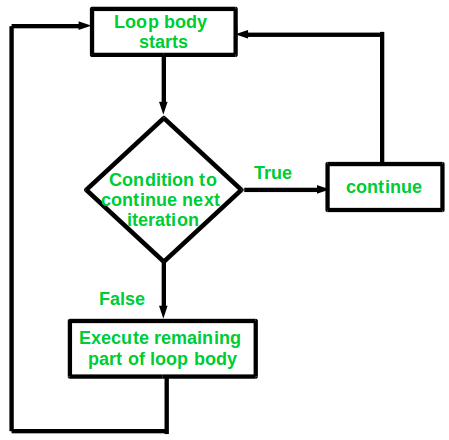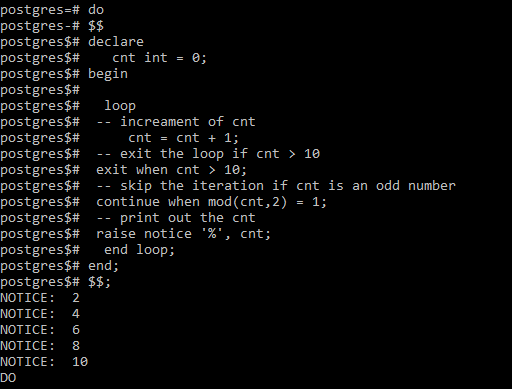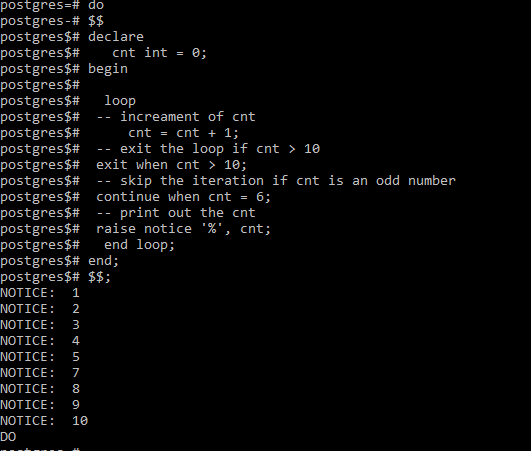# PostgreSQL – Continue

• Last Updated : 20 Sep, 2021

In PostgreSQL ,The continue statement is used to skip the current iteration prematurely and directly move on to the next iteration. The continue statement is used in conjecture with all types of loops including unconditional loops, while loops and for loops.Syntax:

`CONTINUE [ label ] [ WHEN boolean-expression ];`

If we analyze the above syntax:

• label: If no label is present, the next iteration of the loop starts. That is, all statements remaining in the loop body are skipped, and control returns to the loop control expression.However, if the label is present, it specifies the label of the loop whose execution will be continued.

• WHEN condition: It is simply a boolean expression that specifies the condition to skip the current iteration of the loop. If the condition is true, then the current loop iteration will be skipped. However, if it is false, the loop follows the normal flow pattern.

Both the label and WHEN condition is optional and may or may not be used with the continue statement;

Example 1 :

The following example will be used to display the even numbers from 1 to 10.

```do
\$\$
declare
cnt int = 0;
begin
loop
-- increment of cnt
cnt = cnt + 1;
-- exit the loop if cnt > 10
exit when cnt > 10;
-- skip the iteration if cnt is an odd number
continue when mod(cnt,2) = 1;
-- print out the cnt
raise notice '%', cnt;
end loop;
end;
\$\$;```

Output:In the above example, we use the continue statement to skip the odd numbers by using the fact that the remainder when an odd number is divided by 2 is 1.

#### Example 2 :

The following example will be used to display all numbers from 1 to 10 without displaying the number 6.

```do
\$\$
declare
cnt int = 0;
begin
loop
-- increment of cnt
cnt = cnt + 1;
-- exit the loop if cnt > 10
exit when cnt > 10;
-- skip the iteration if cnt is an odd number
continue when cnt = 6;
-- print out the cnt
raise notice '%', cnt;
end loop;
end;
\$\$;```

Output:In the above example, we use the continue statement to skip the iteration when the value of the cnt variable reaches 6.

My Personal Notes arrow_drop_up